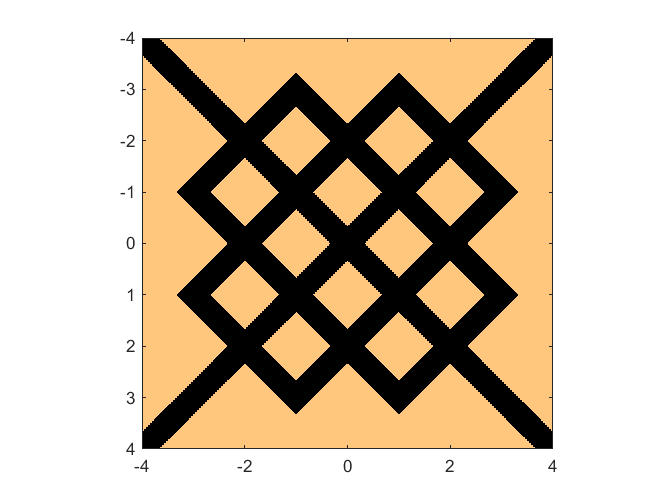An Ornamental Geometric Inequality

I came across this "ornamental geometric inequality" in a tribute to Lothar Collatz.

Contents

An Inequality

I mentioned the German mathematician Lothar Collatz in my post in January on his 3n+1 Conjecture. Here is a beautiful inequality from his 1934 paper titled "Ornamental Geometric Inequalities". Consider the subregion of $-4 \le (x,y) \le 4$ for which

$$||||x| - 1| - 1| - |||y| - 1| - 1|| \ge \frac{1}{3}$$

I've never seen four absolute value signs in a row before.

Let's visualize it with MATLAB.

[x,y] = meshgrid(-4:1/256:4);
z = abs(abs(abs(abs(x)-1)-1) - abs(abs(abs(y)-1)-1)) >= 1/3;
imagesc([-4,4],[-4,4],z);
axis image
colormap(copper)References

Lothar Collatz, 1910-1990, <http://www.math.uni-hamburg.de/home/collatz/auflage3-beta2.pdf>

Lothar Collatz, Gleichungen geometrischer Ornamente, (Kurvengleichungen mit Absolutstrichen). Z. mathem. u. naturw. Unterr. 64 (1934), 165-169.

Published with MATLAB® R2014b

|

댓글

댓글을 남기려면 링크 를 클릭하여 MathWorks 계정에 로그인하거나 계정을 새로 만드십시오.